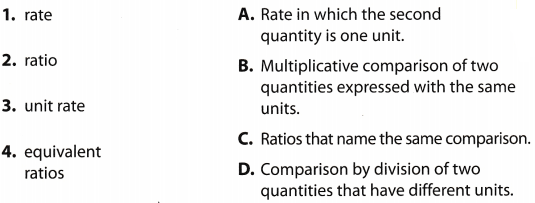# Texas Go Math Grade 6 Module 7 Answer Key Representing Ratios and Rates

Refer to our Texas Go Math Grade 6 Answer Key Pdf to score good marks in the exams. Test yourself by practicing the problems from Texas Go Math Grade 6 Module 7 Answer Key Representing Ratios and Rates.

## Texas Go Math Grade 6 Module 7 Answer Key Representing Ratios and Rates

Write each fraction in simplest form.

Question 1.
$$\frac{6}{9}$$
List the factors of 6 and 9.
Then circle the common factors.Question 2.
$$\frac{4}{10}$$
List the factors of 4 and 10.
Then circle the common factors.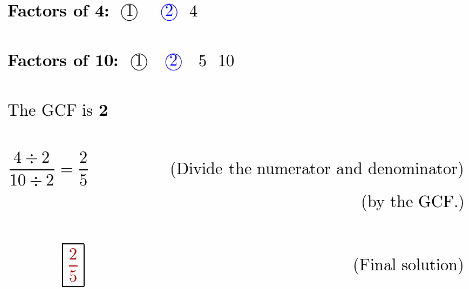Question 3.
$$\frac{15}{20}$$
Simplify $$\frac{15}{20}$$
List the factors of 15 and 20.
Then circle the common factors.Question 4.
$$\frac{20}{24}$$
Simplify $$\frac{20}{24}$$
List the factors of 20 and 24.
Then circle the common factors.Question 5.
$$\frac{16}{56}$$
Simplify $$\frac{16}{56}$$
List the factors of 16 and 56.
Then circle the common factors.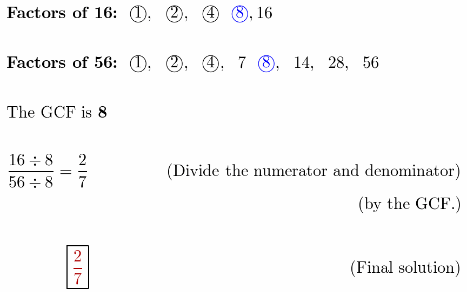Question 6.
$$\frac{45}{72}$$
Simplify $$\frac{45}{72}$$
List the factors of 45 and 72.
Then circle the common factors.Question 7.
$$\frac{18}{60}$$
Simplify $$\frac{18}{60}$$
List the factors of 18 and 60.
Then circle the common factors.Question 8.
$$\frac{32}{72}$$
Simplify $$\frac{32}{72}$$
List the factors of 32 and 72.
Then circle the common factors.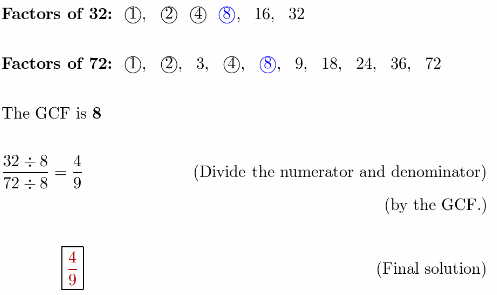Write the equivalent fraction.

Question 9.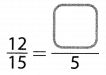Switch sidesQuestion 10.Switch sides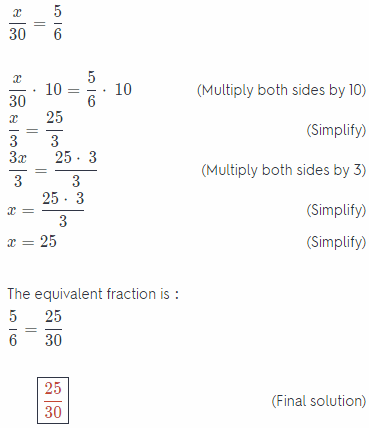Question 11.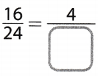Solution to this example is given below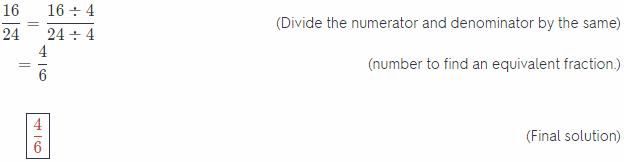Question 12.Solution to this example is given belowQuestion 13.Solution to this example is given belowQuestion 14.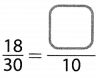Solution to this example is given belowQuestion 15.Solution to this example is given below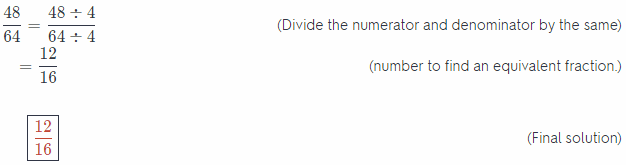Question 16.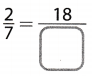Given expression
$$\frac{2}{7}=\frac{18}{x}$$

Multiply both sides of the equation with  to isolate the variable on 1 side of the equation:
$$\frac{2}{7} \times \frac{7 x}{2}=\frac{18}{x} \times \frac{7 x}{2}$$
Evaluate:
x = 63
Therefore the equivalent fraction is:
$$\frac{2}{7}=\frac{18}{63}$$

Visualize Vocabulary
Use the ✓ words to complete the chart. Choose the review words that describe multiplication and division.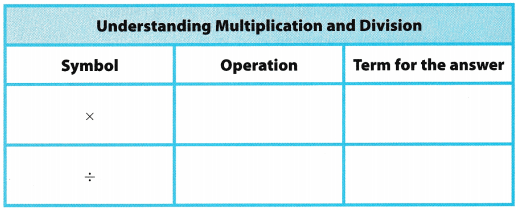Understand Vocabulary

Match the term on the left to the definition on the right.